# Ten dices

When you hit ten dices at the same time you get average 35.
How much do you hit if every time you get six, you're throwing the dice again?

x =  42

### Step-by-step explanation:

x = 35 + 1/6 x

x = 35 + 1/6•x

5x = 210

x = 42

Our simple equation calculator calculates it.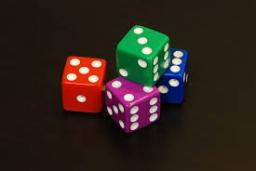Did you find an error or inaccuracy? Feel free to write us. Thank you!Math student
waht the hallMath student
oh nv mind i got tisTips to related online calculators
Do you have a linear equation or system of equations and looking for its solution? Or do you have a quadratic equation?
Would you like to compute count of combinations?

## Related math problems and questions:

• Six on diceWhat is the probability that when throwing two dice will fall at least one six?
• Dice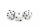How many times must throw the dice, the probability of throwing at least one six was greater than 90%?
• Fall sum or sameFind the probability that if you roll two dice, it will fall the sum of 10, or the same number will fall on both dice.
• Population growth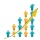How many people will be on Earth from two people for 5,000 years, if every couple has always 4 children, (2 boys and 2 girls) at the age of 25-35, and every man will live 75 years?
• Dices throwsWhat is the probability that the two throws of the dice: a) Six falls even once b) Six will fall at least once
• Pilsen circus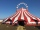The arrival of the circus in Pilsen was seen in the morning at 08:00 by a city citizen. He passed this information on 08:15 to three other residents of the city. Each of these three people then informed the other three residents at 08:30, and again at 08:
• Infinite decimalImagine the infinite decimal number 0.99999999 .. ... ... ... That is a decimal and her endless series of nines. Determine how much this number is less than the number 1. Thank you in advance.
• DicesWe will throw two dice. What is the probability that the ratio between numbers on first and second dice will be 1:2?
• Three dice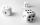When you throw three dice was the sum total of the dice 10. The yellow dice fell one eye more than on the red and brown fell 3 eyes less than red. How many eyes fell on every dice?
• The diceWhat is the probability of events that if we throw a dice is rolled less than 6?
• SavingsSuppose on your 21st birthday you begin making monthly payments of \$500 into an account that pays 8% compounded monthly. If you continue the payments until your 51st birthday (30 years), How much money is in your account? How much of it is interesting? Sh
• Saving per cents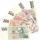The first day I save 1 cent and every next day cent more. How many I saved per year (365 days)?
• Sum of fall dices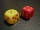What is the probability that the sum of 9 will fall on a roll of two dice? Hint: write down all the pairs that can occur as follows: 11 12 13 14 15. . 21 22 23 24. .. . 31 32. .. . . . . . .. . 66, count them, it's the variable n variable m: 36, 63,. .. .
• Roll the diceWhat is the probability that if we roll the dice, a number less than 5 falls?
• Monty Hall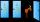Suppose you're on a game show, and you're given the choice of three doors: Behind one door is a car; behind the others, goats. You pick a door, say No. 1, and the host, who knows what's behind the doors, opens another door, say No. 3, which has a goat. Wh
• Three dice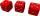What is the probability that when we roll three dice, we roll the numbers 1,2,3?
• Ships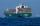Red ship begins its circuit every 30 minutes. Blue boat begins its circuit every 45 minutes. Both ships begin their sightseeing circuit in the same place at the same time always at 10:00 o'clock. a / What time does meet boat again? b / How many times a da